# Measuring the Thickness of Thick Films with a Spectroscopic Reflectance System

The MPROBE® can be used to precisely measure almost any translucent film with a thickness between 1 nm and 1 mm, making it suitable in most thin film applications.

The data from the system can be measured using two different methods, which are model/curve fitting, or Fast Fourier Transform (FFT) analysis on the captured spectrum. Generally, model fitting is used for thinner films (between 1 nm and 1 µm in width) and FFT is used for films of a thickness greater than 20 µm.

Within the intermediate thickness range (1 µm to 20 µm), the use of either method is appropriate, with the preference depending on the experimental requirements.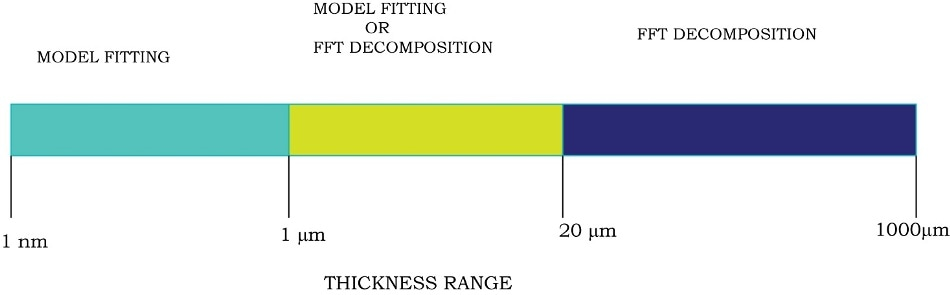Figure 1. Data analysis method depending on the thickness range.

Using the Fast Fourier Transform (FFT) method can be preferential, as it has low calibration requirements (variations in the intensity have no impact on the results), the analysis happens quickly, and a minimal amount of prior information on the sample is needed.

However, it is not a perfect method. FFT requires the refractive index (R.I.) information of the materials to be known and, until recently, has provided a lower accuracy than a curve fitting method. The accuracy of FFT has been improved by new additions to Semiconsoft’s TFCompanion software, which now has an enhanced decomposition algorithm. Following these improvements, the method can now deliver accuracies better than 0.1%.

## Thickness Range of the Practical FFT Use

FFT exploits the periodicity of interference between the wavelength and intensity, a phenomenon known as interference fringes. The material’s R.I. and the wavelength range of the measurement determine the minimum thickness of a film, which can be measured accurately using FFT.

This is because sampling only one period, or a fraction of a period’s function, (as would happen for very thin films) results in poor FFT data. The measurement error of FFT methods for different wavelength ranges and the thicknesses are given in Table 1.

Table 1. FFT measurement errors.

Thickness/
Wavelength range
200
nm
500
nm
700
nm
1,000
nm
400-1,000 nm Error(absolute value) 92 nm 36 nm 6 nm 0.6 nm
Error (%) 46% 7% 0.9% 0.06%
400-1,700 nm Error (absolute value) 49 nm 2 nm 1.2 nm 0.4 nm
Error (%) 24% 0.4% 0.017% 0.04%

A Fast Fourier Transform method was used to analyze simulated reflectance spectra for oxide films with widths of 200 nm, 500 nm, 700 nm and 1,000 nm, and the absolute and relative measurement errors were calculated. Spectra were then calculated for the measurement ranges 400 – 1,700 nm and 400 – 1,000 nm.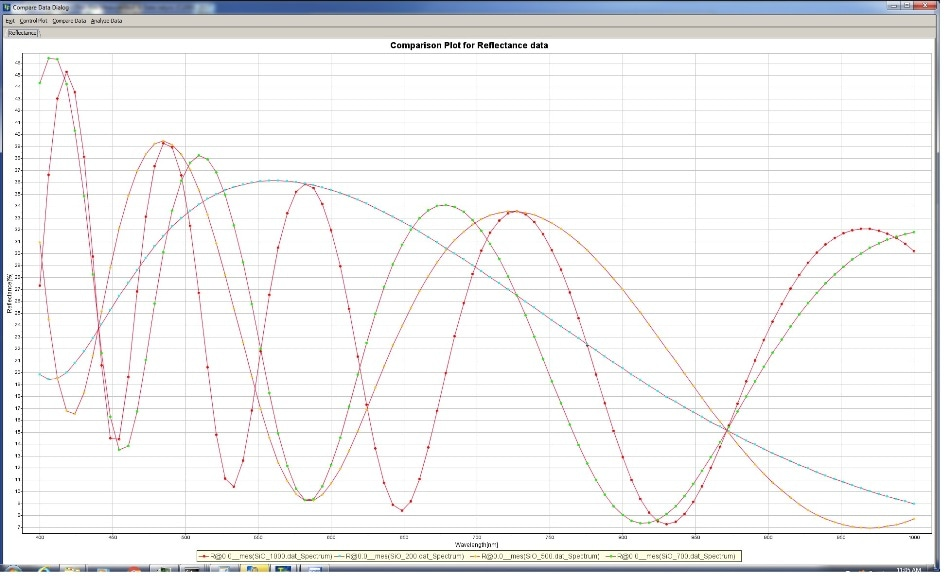Figure 1. Simulated reflectance spectrum (wavelength range: 400-1,000 nm) of the SiO2/Si. Oxide thicknesses: 200 nm, 500 nm, 700 nm and 1,000 nm (Oxide thickness corresponding to one period in visible range is ~ 320 nm)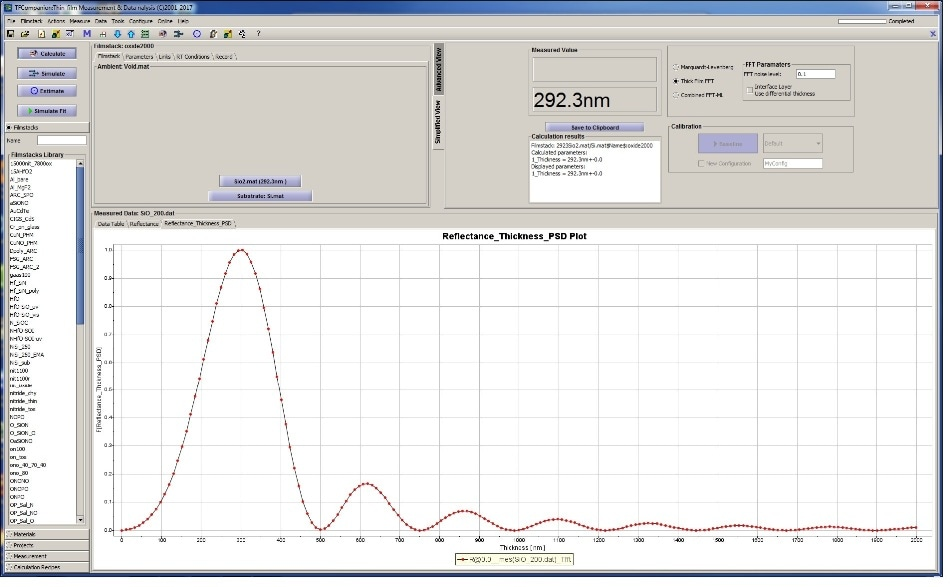Figure 2. Thickness of the 200nm oxide determined using FFT (400-1,000 nm wavelength measurement range). A large thickness measurement error (92 nm) shows that FFT maybe not practical for this thickness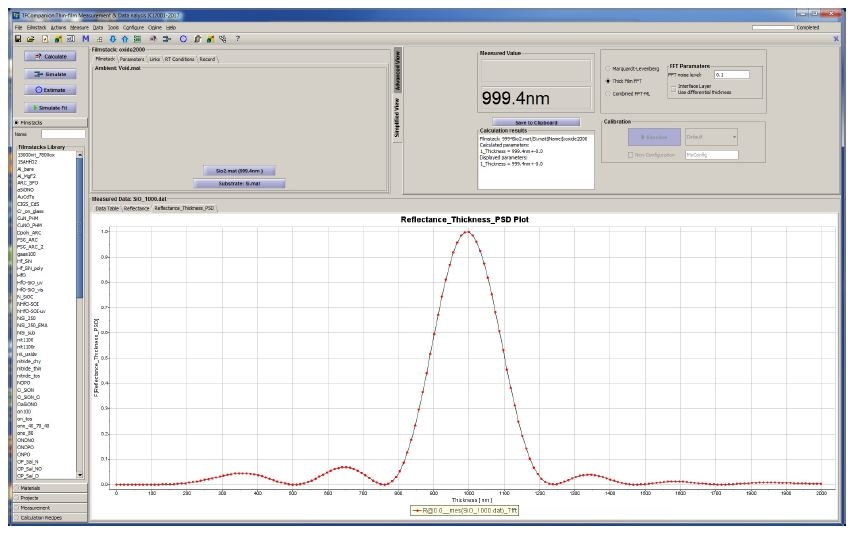Figure 3. Thickness of the 1000 nm oxide determined using FFT (400-1,000 nm wavelength measurement range). A very small measurement error (0.6 nm) shows that FFT can be used successfully for this thickness

## Applications of FFT Analysis

### Analyzing Multilayer Polymer Films

Multilayer Polymer Films have a wide application base, being used in a multitude of ‘everyday’ products, such as stickers and food packaging.

In this example, a 4-layer polymer film, used as a sticker, is analyzed. The film has a structure of separator/silicon/adhesive/PET (substrate). The silicon layer is a thin film, of thickness less than 1 µm, which can be peeled off to leave the PET film naked. This then behaves as an adhesive.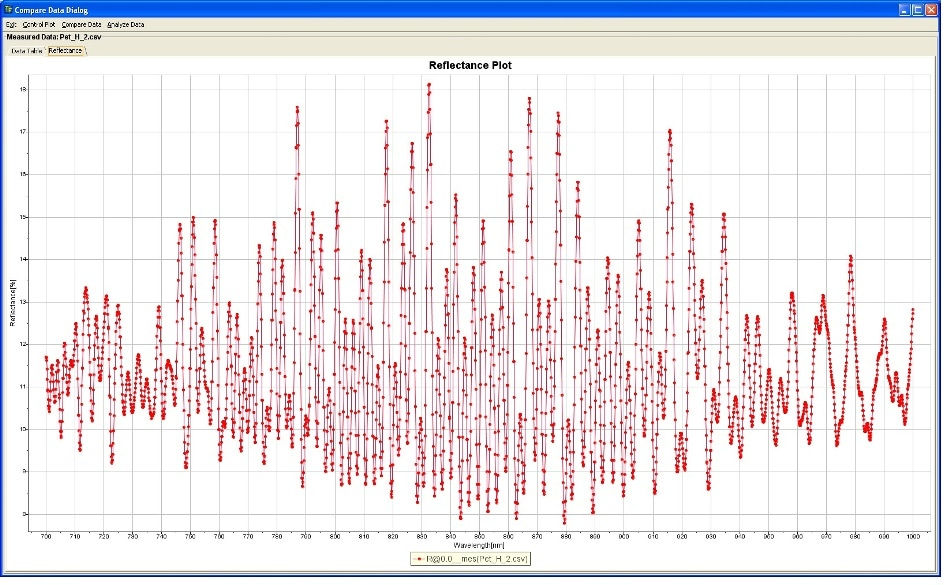Figure 4. Reflectance spectrum measured with MProbeVisHR (700 nm -1,000 nm wavelength range). Sample 1 is a 4 layer polymer web (sticker).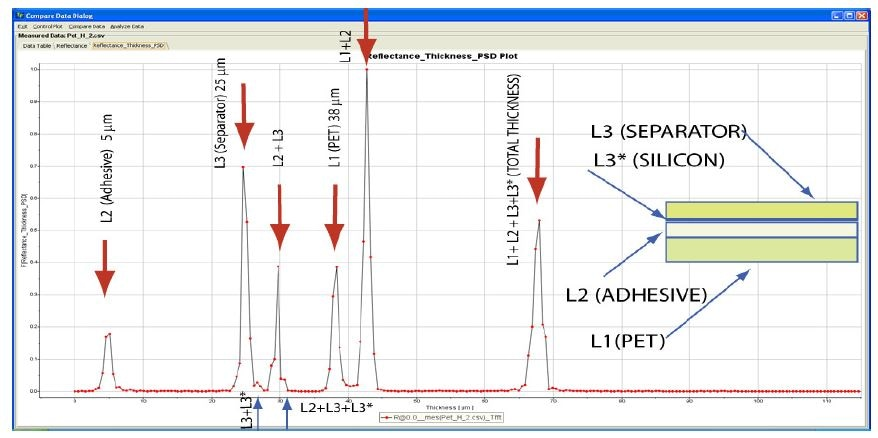Figure 5. FFT decomposition data analysis of the data (Fig. 4). Position of the peaks indicate the thickness of the layers.

Running a Fast Fourier Transform analysis results in a peak for every interface pair, which also correspond to layers in the multilayer film. The height of the peak can be used to elucidate information about the interface’s quality, whereas the peak position can be used to determine the layer’s thickness.

As a peak is given for every interface, a three-layer stack should give an FFT spectrum with six peaks, with the final peak relating to the total thickness of the stack. Figure 5 shows an example of this.

In Figure 5, the silicon layer (peak 3*) has too low a thickness (less than 1 µm) to be seen individually, and instead, is combined with the separator + adhesive, and the separator layers. The low thickness of the silicon layer means that the total thickness peak (peak 6) is also broader.

Using TFCompanion software allows researchers to automatically determine each peak and match them to corresponding layers. This is easier for the software if the structure of the film being measured is known with the approximate thicknesses of each layer, which helps each layer to be correctly identified.

If this information is not known, it can often be determined from the FFT data and then inputted back into the model.

Figure 5 shows the FET decomposition spectrum with all of the layers shown, with the exception of silicon. In cases where the membrane is too thin, layers can be hidden by other peaks, or the peak can be too low to identify.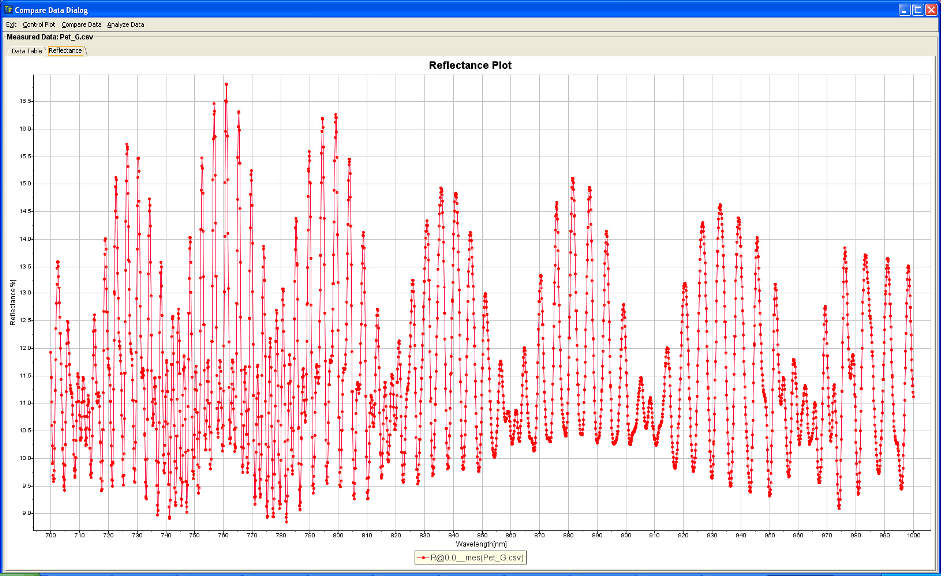Figure 6. Reflectance spectrum measured with MProbeVisHR (700 nm – 1,000 nm wavelength range). Sample 2 is a 4 layer polymer web (sticker). Sample 2 has the same structure as Sample 1 but, in this case, the thicknesses of the Separator and PET (Substrate) layers are similar.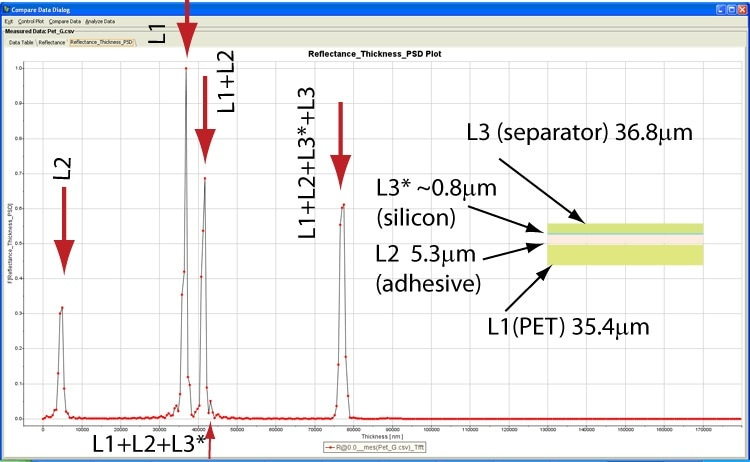Figure 7. FFT decomposition data analysis of the data (Fig. 6).

Deconvolution of the FFT data from Sample 2 (as shown in Figure 7) does not show Layer 3, however, Layer 3’s impact can be observed in the broadening of Layer 1’s peak. Whilst the peak of L1 could be deconvoluted to give the thickness of L3, it is more practical to use the information provided on total thickness and the thickness of L1 and L2, to determine the thickness of L3.

Figure 8 shows how the missing thickness of a layer (e.g. L3) can be found from the total thickness, using the software’s different thickness option.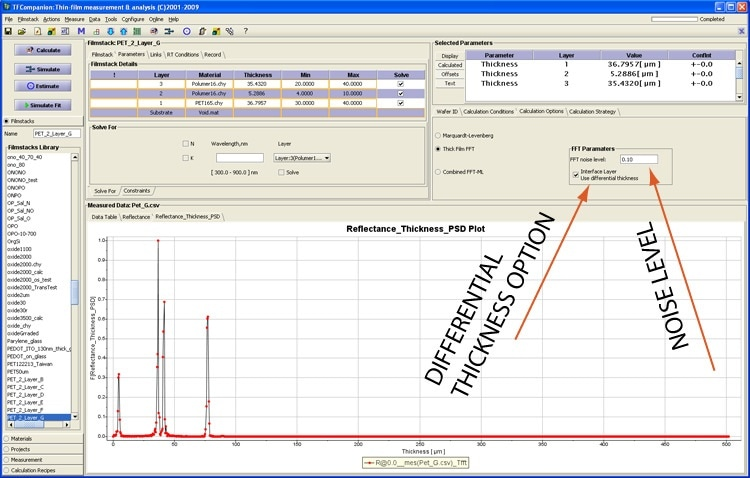Figure 8. Setting differential thickness option to determine thickness of the “hidden” layer/interface from the total thickness. Noise level setting helps separate Layer thickness data from the noise.

### Measuring an Alumina (Sapphire) Layer

Thick layers such as alumina, yttrium oxide and parylene all have a refractive index which is highly dependent upon the layers deposition conditions. As the R.I. can be different between samples, using data from a library or other optical properties databases alongside the FFT method can give erroneous data.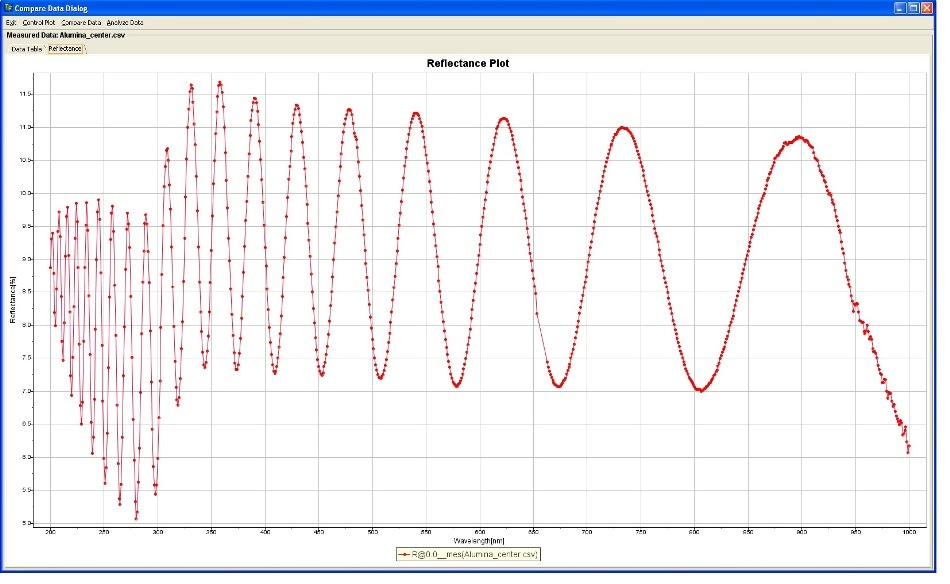Figure 9. Reflectance of Alumina on glass measured with MProbe UVVisSR system (wavelength range: 200 nm -1,000 nm)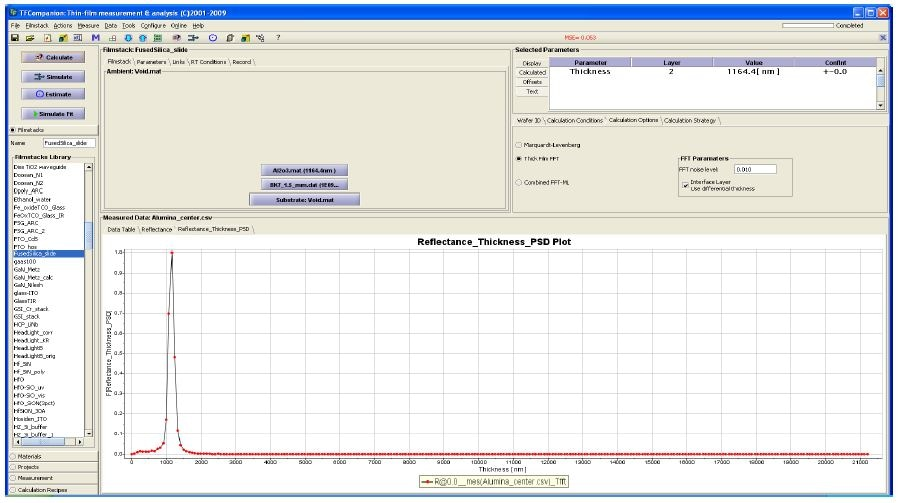Figure 10. FFT decomposition of the data on Fig. 8. using library Alumina material properties. The peak position indicates the thickness of 1,164 nm

Directly comparing the library data model against measured data using an FFT method demonstrates that the R.I. value given in the library data is not correct.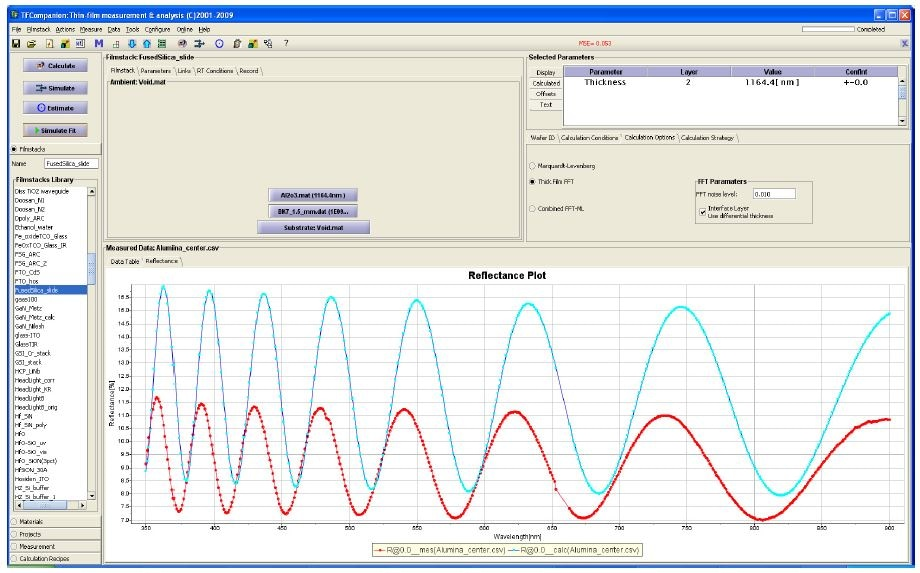Figure 11. Direct comparison of the FFT results model (red) and measured data (blue) shows that R.I. is not correct.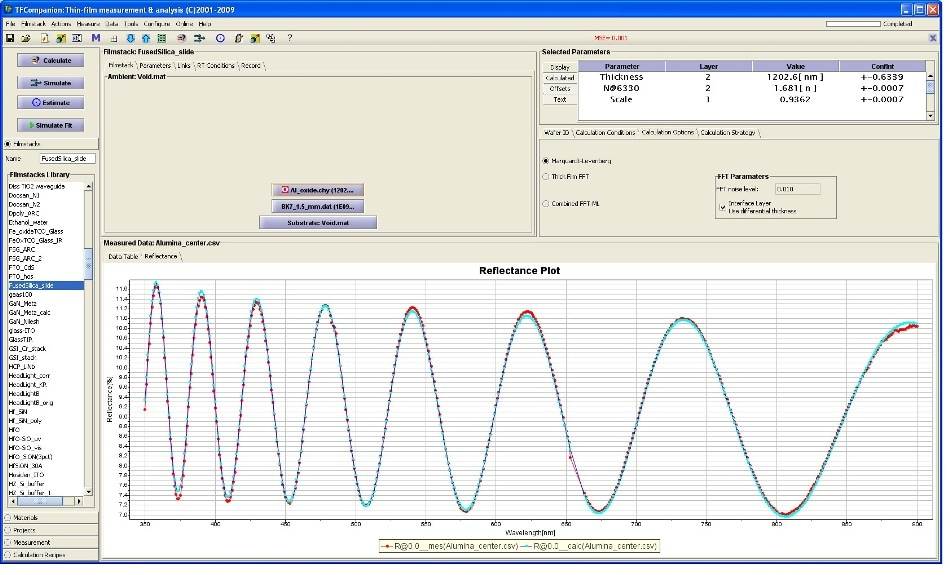Figure 12. Direct fit of the model to measured data to determine the thickness and R.I. of alumina. Thickness 1,202 nm.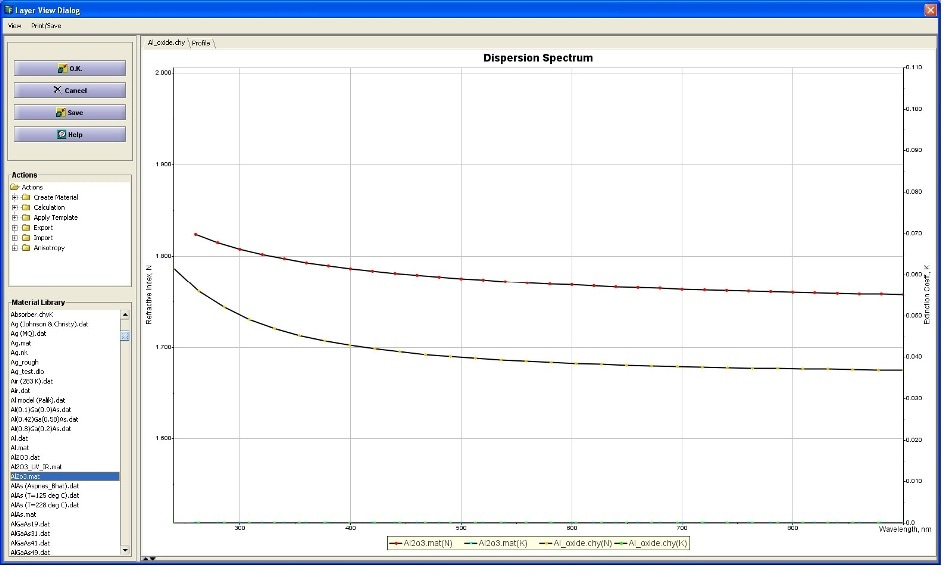Figure 13. Alumina R.I. determine from the measurement (yellow) and library alumina R.I. (red)

### Measuring Hard Coatings

Hard coatings are an essential part of many systems, such as the polycarbonate surfaces used in automotive lighting covers and eyeglass lenses. When a hard coating is deposited on a surface, it penetrates into the carbon, creating an Inter Penetration Layer (IPL) which is relatively thin (approx. 1 μm).

When measuring hard coatings for QC, it is important that the thickness of both the hard coating layer and the IPL are measured.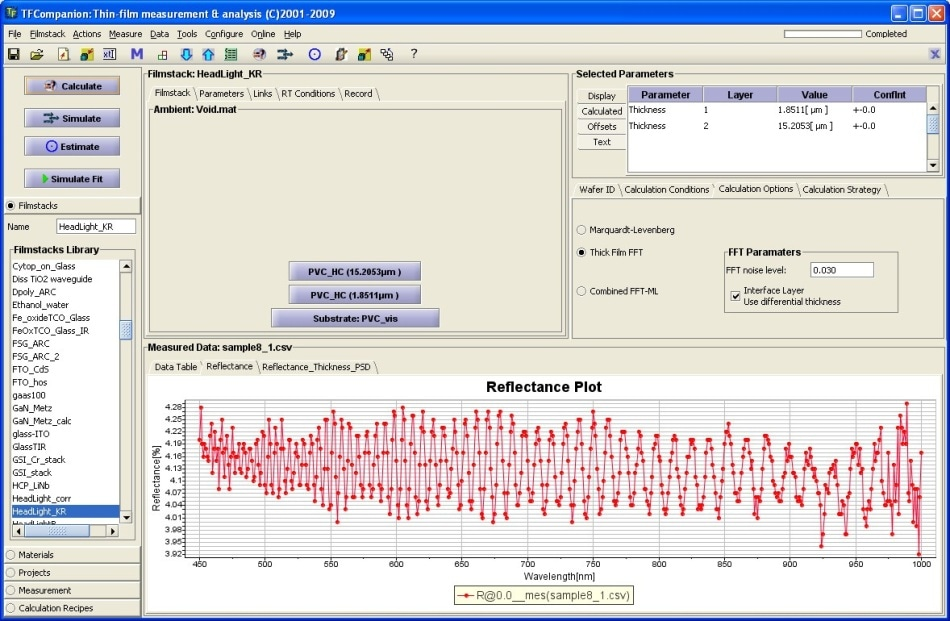Figure 14. Reflectance spectra of polycarbon with hardcoat sample (headlight). Measurement is taken with MProbe Vis system (wavelength range: 400-1,000 nm)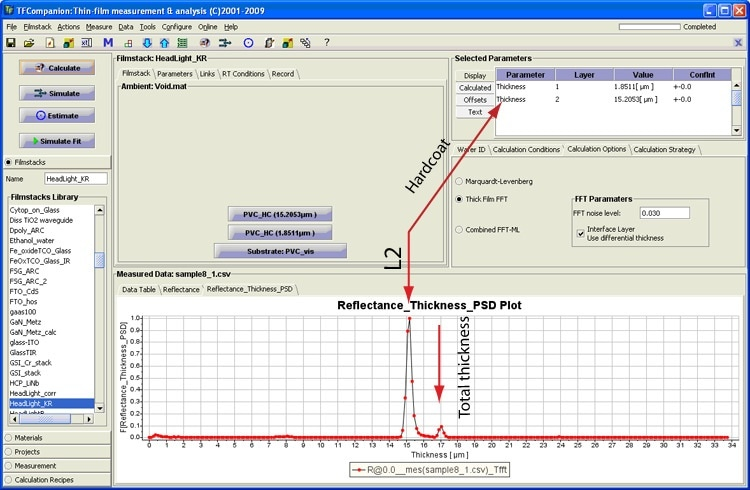Figure 15. FFT decomposition of the measurement data (Fig. 14). A differential thickness option is activated and IPL is determined from total thickness. Hard coat: 5.2 μm, IPL: 1.85 μm

The IPL layer exhibits a refractive index which is between the R.I. values of the hard coating and the polycarbonate layer, meaning there is a weak optical contrast between the two different materials.

This means that the peak from the IPL is very weak. However, this issue can be avoided by running a differential IPL thickness determination (from the total thickness) to give more accurate results.

## Improving the Accuracy of FFT Analysis

When using an FFT method, the overall accuracy of the thickness measurement is dependent on how accurately locating the peak position. The error of this measurement can be given using the equation: (Xn+1-Xn)/2, where, as in Figure 1, Xn and Xn+1 are points either side of the peak.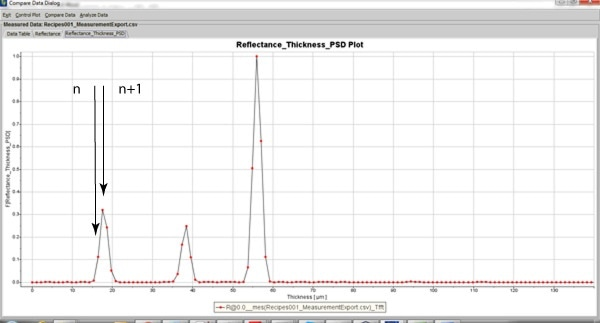Figure 16. FFT points resolution. Distance between adjacent FFT points (bins) determines maximum error.

Several options are available, using TFCompanion software, to improve the accuracy of analysis, which include:

1. Interpolating between data points
2. Fit a Gaussian function to the peak to determine the position
3. Increasing the number of data points
4. Filter the data (apodization) – indirectly improves accuracy by removing aliases and additional peaks. Especially helpful for noisy data.

Below, these different methods are used with TFCompanion for the measurement of a polymer film.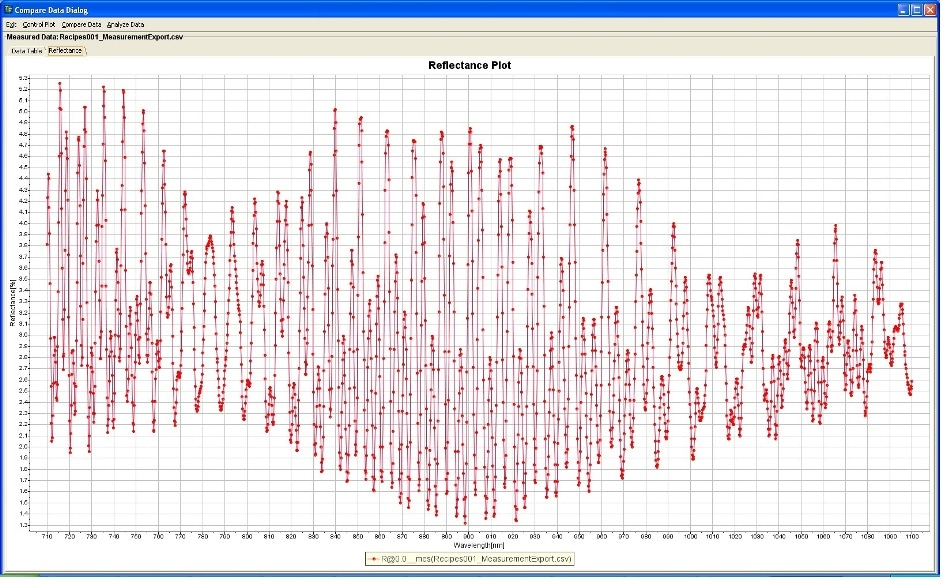Figure 17. Measured reflectance spectrum of coating (~ 18 µm) on 38 µm PET

## Interpolating Between Points and Applying a Gaussian Fit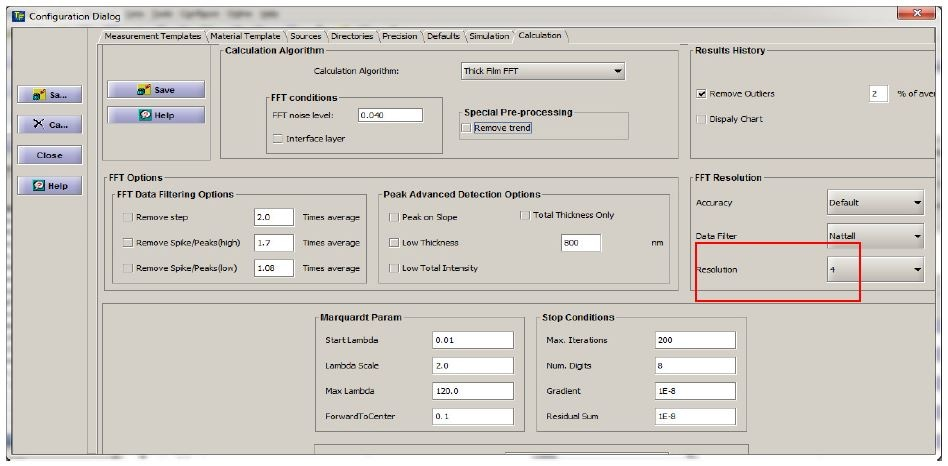Figure 18. Selecting accuracy level.

TFCompanion allows for three different modes of accuracy:

• Maximum accuracy – A full Gaussian curve is fitted to the peak
• High accuracy – Interpolation between the points using a Gaussian model
• Default – No fitting or interpolation

## Increasing the Number of Data Points

The data points from FFT analysis are spread over the greatest range of the thickness measurement for which data can be gathered. However, in most cases, only a section of this range is of interest. E.g. in this example, the sample thicknesses are only 18 µm and 38 µm whereas the thickness range of measurement is 280 µm.

The number of data points in the thickness range of interest can be increased using a technique called oversampling.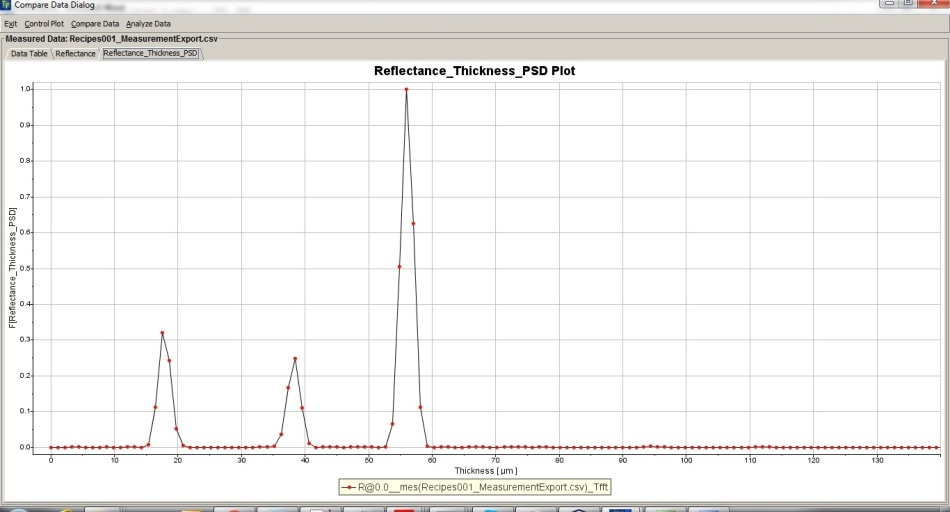Figure 19. Resolution improvement by using increased number of FFT points. 1 is default resolution, 5 – maximum resolution.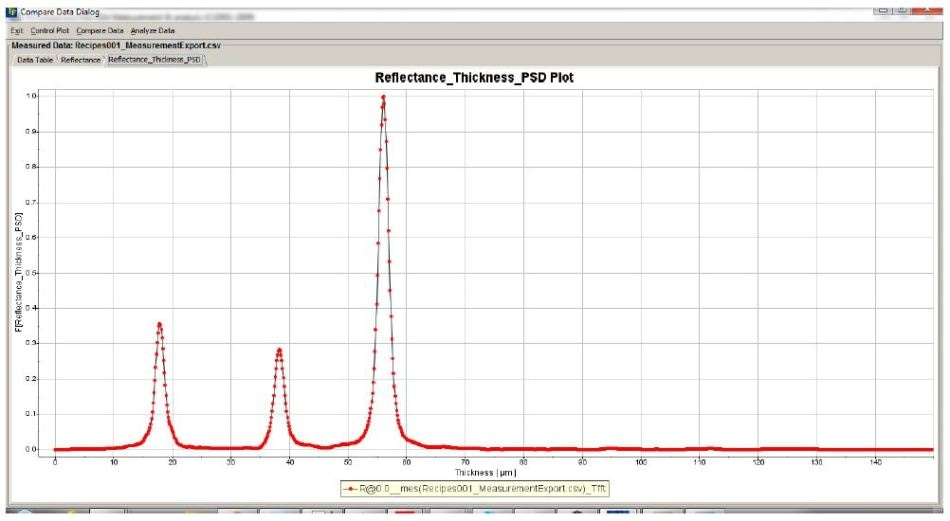Figure 20. Peaks using default resolution (1)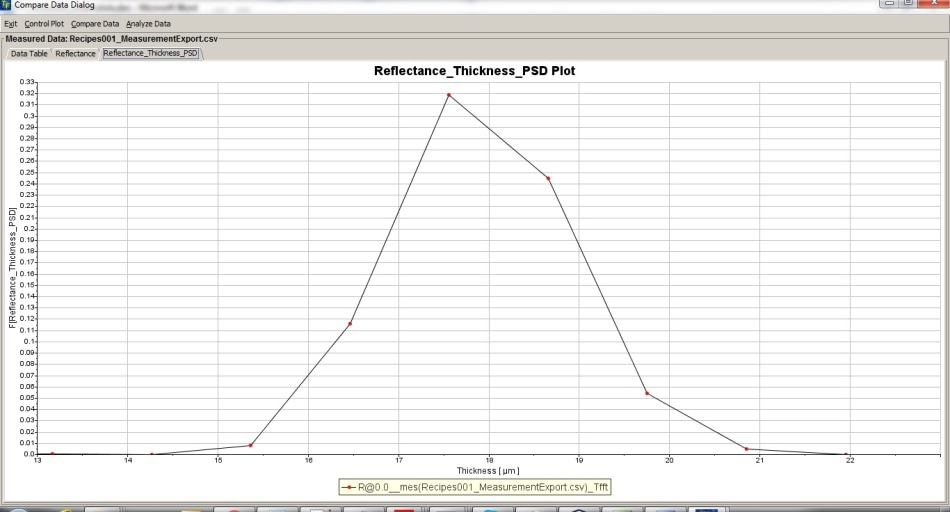Figure 21. Peaks using high resolution (4)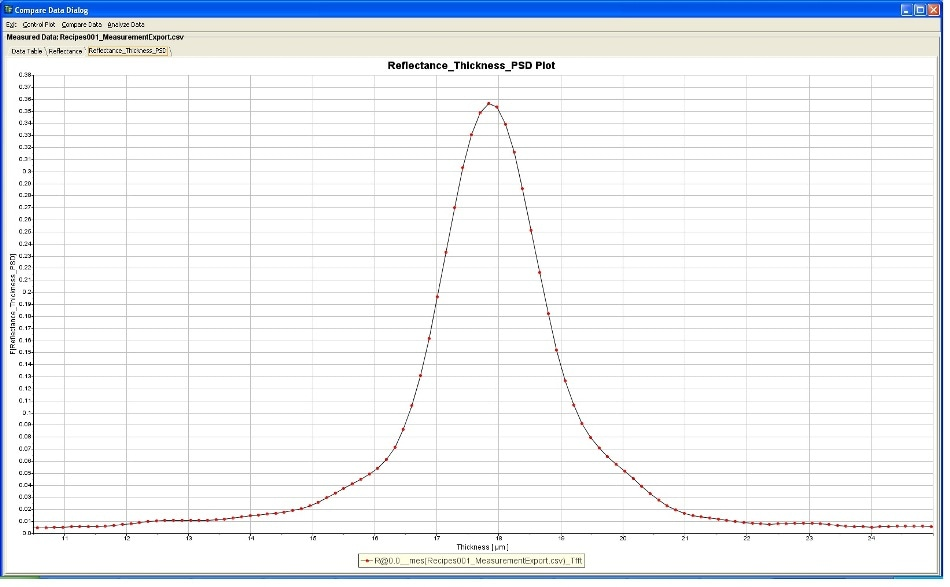Figure 22. Peak detail from fig. 20 (default resolution - 1)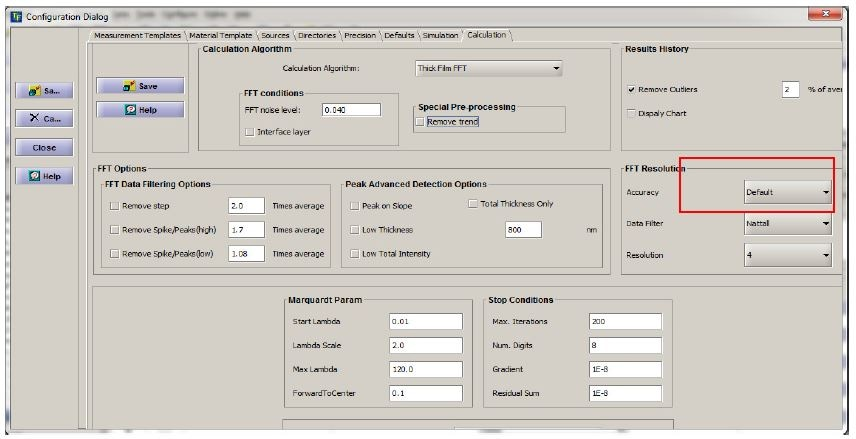Figure 23. Peak detail from Fig. 20 (high resolution - 4)

Table 2. Maximum possible absolute measurement error depending on the resolution for this measurement

Resolution 1 2 3 4
Maximum possible error (µm) 0.548 0.274 0.137 0.068

## Filtering the Data (Apodization)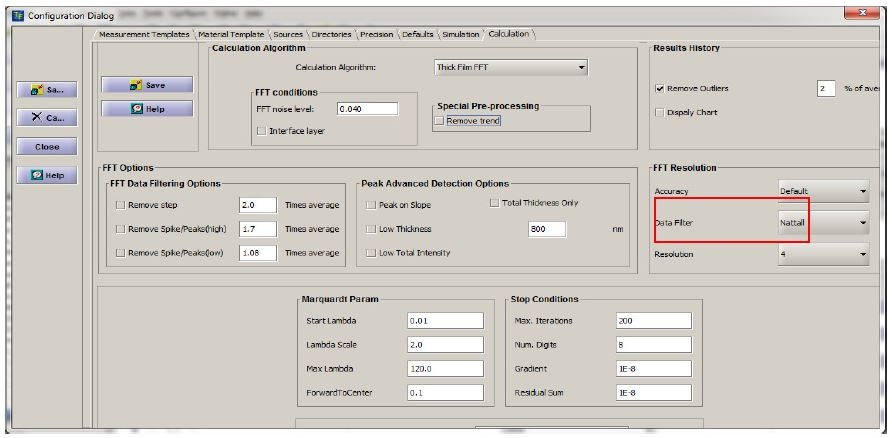Figure 24. Apodization filter selection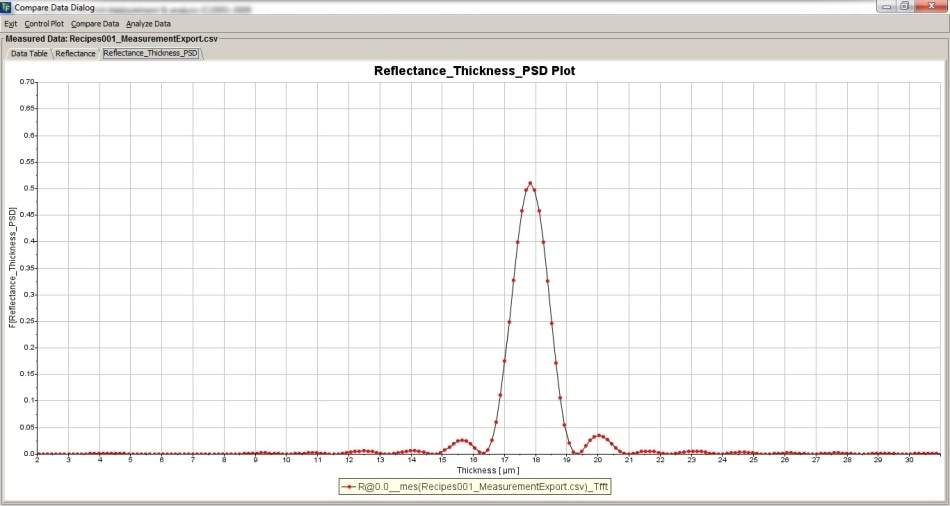Figure 25. Peak without data apodization/ Two aliases are visible.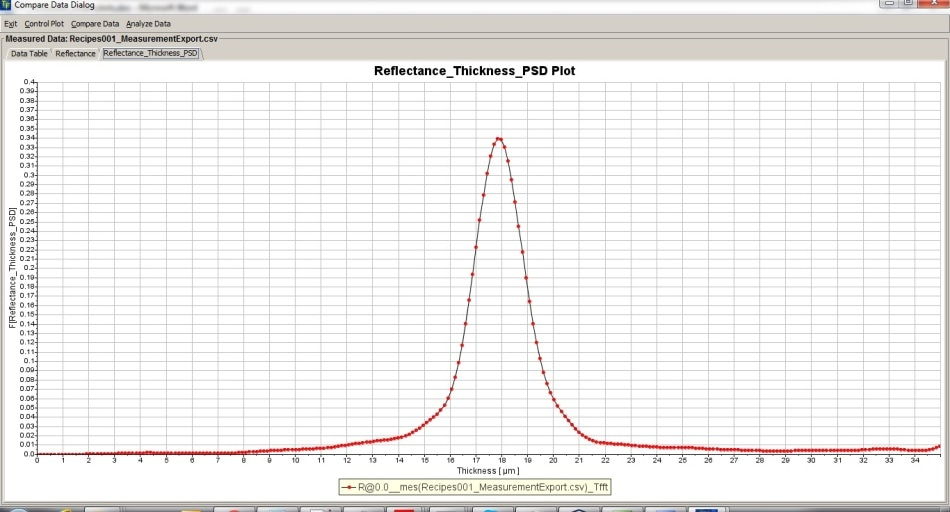Figure 26. Peak with data apodization (peak is the same as on Fig. 25)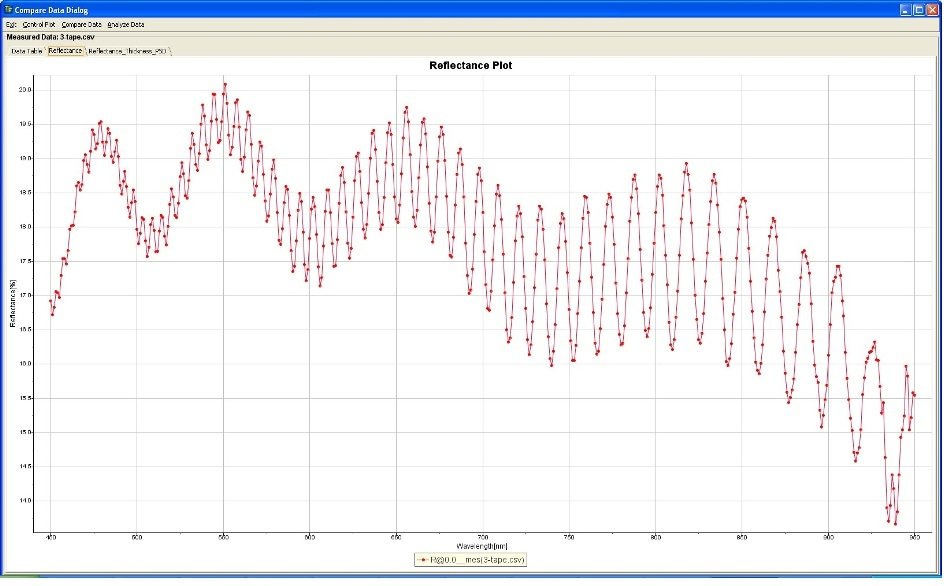Figure 27. Reflectance spectrum of GaN on sapphire (400-1000 nm wavelength range)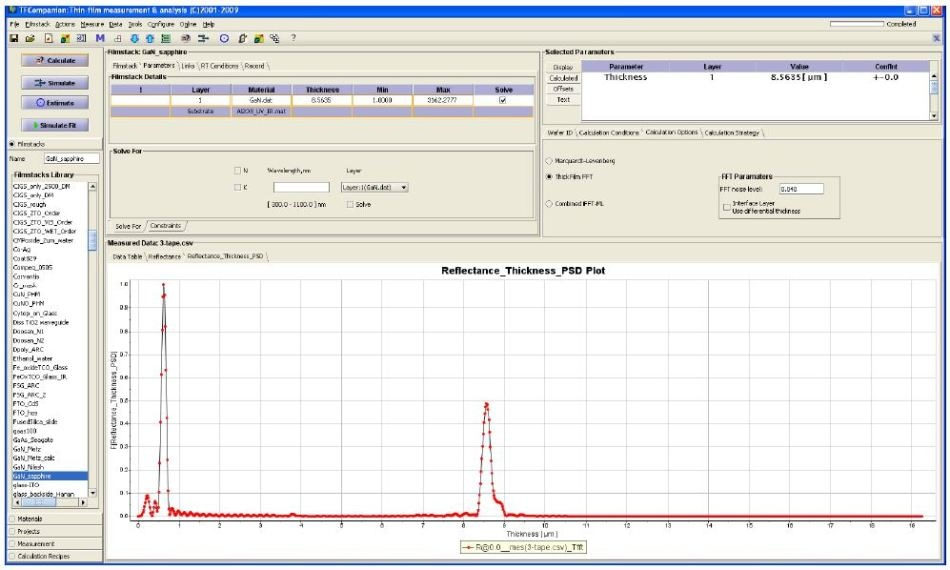Figure 28. Measurement of GaN (fig. 27) without data apodization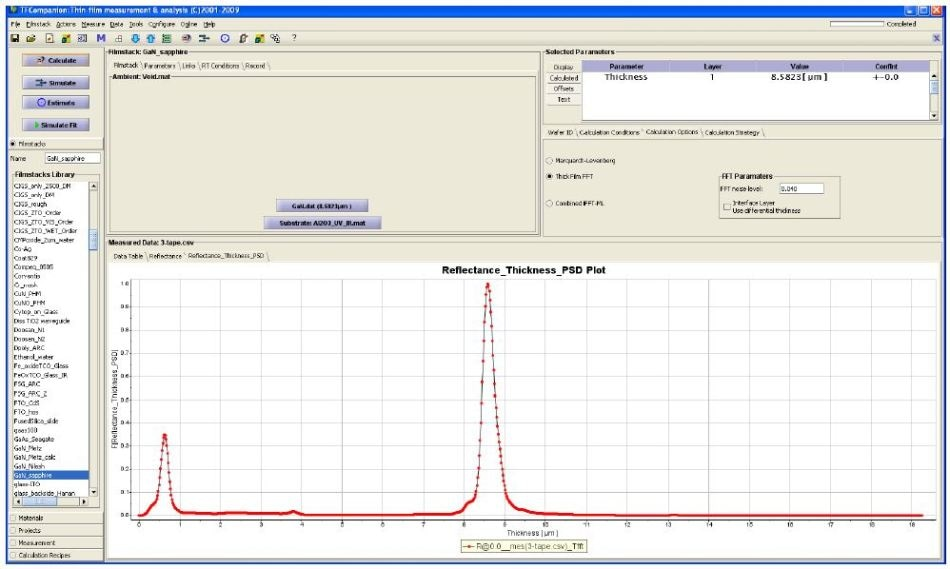Figure 29. Measurement of GaN (Fig. 27) with data apodization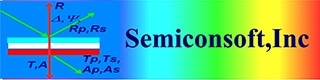This information has been sourced, reviewed and adapted from materials provided by SemiconSoft.

## Citations

• APA

SemiconSoft. (2018, October 25). Measuring the Thickness of Thick Films with a Spectroscopic Reflectance System. AZoM. Retrieved on October 30, 2020 from https://www.azom.com/article.aspx?ArticleID=16814.

• MLA

SemiconSoft. "Measuring the Thickness of Thick Films with a Spectroscopic Reflectance System". AZoM. 30 October 2020. <https://www.azom.com/article.aspx?ArticleID=16814>.

• Chicago

SemiconSoft. "Measuring the Thickness of Thick Films with a Spectroscopic Reflectance System". AZoM. https://www.azom.com/article.aspx?ArticleID=16814. (accessed October 30, 2020).

• Harvard

SemiconSoft. 2018. Measuring the Thickness of Thick Films with a Spectroscopic Reflectance System. AZoM, viewed 30 October 2020, https://www.azom.com/article.aspx?ArticleID=16814.

## Tell Us What You Think